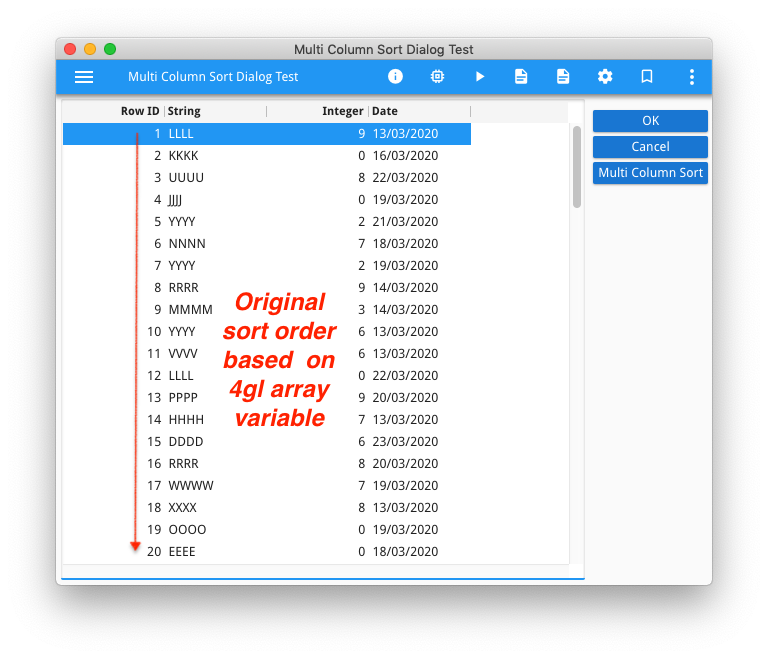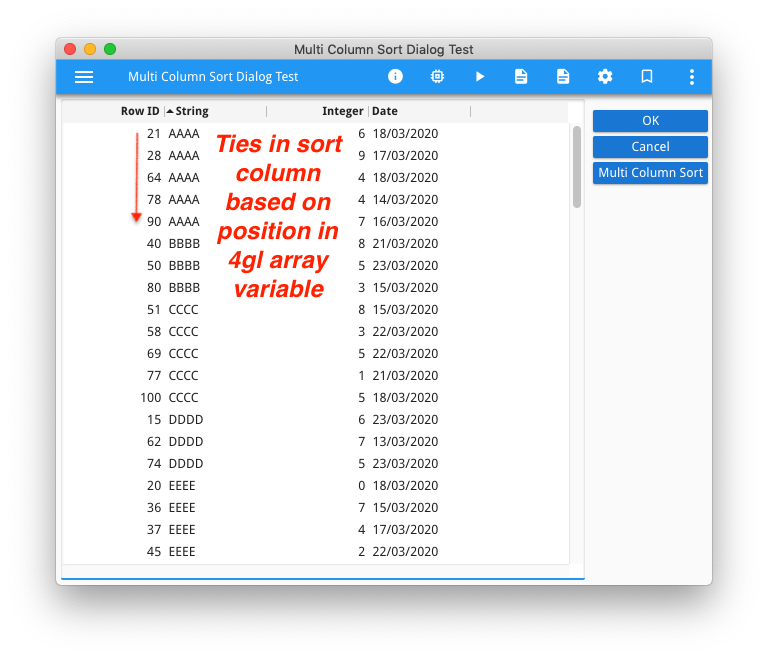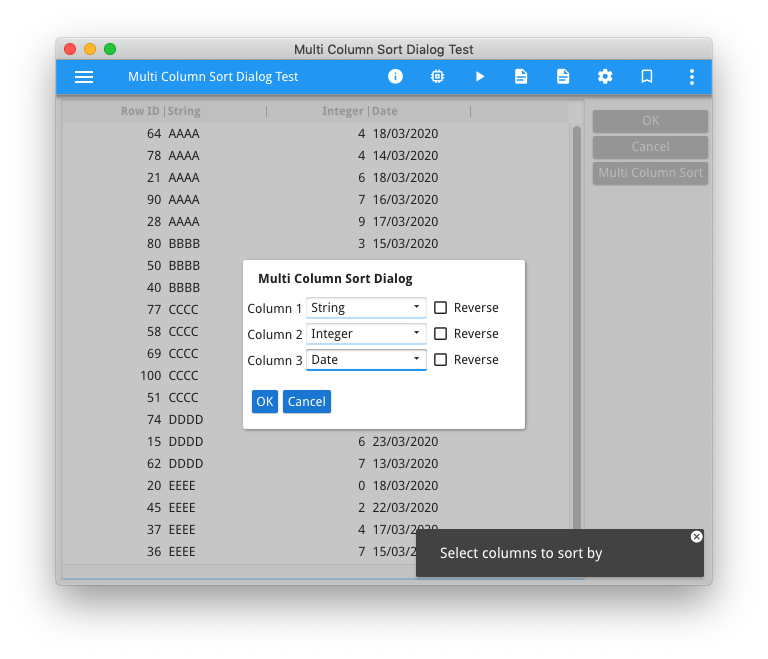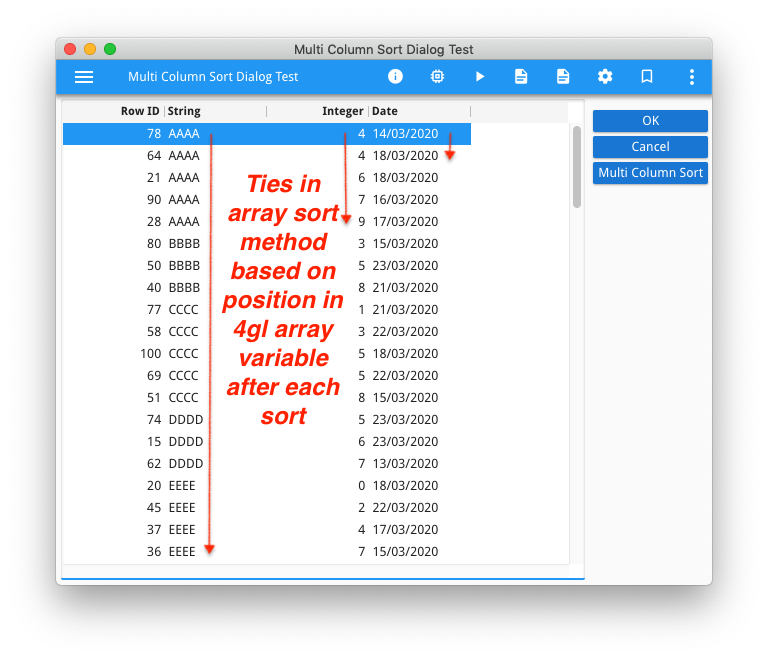# Multi-Column SortingHow to sort on two or more columns?

How are ties broken when sorting?

To answer this question, I will make use of the fgl_multicolumnsortdialog repository available from our repository on GitHub.

A multi column sort is where you want the sort order to be based on two or more values.  This could be cases like last name + first name,  year + month, country + region + city, and other cases typically involving codes of various organisational units.

I will first answer the question on how ties are broken.

If you run the test program from the repository, you will note that the original sort order is as per the contents of the array, note the contents are effectively sorted by the contents of the first column which I populated with the array index.Now if you click on a column heading, the array is sorted as per the column heading.  If you look in the column you sorted on for values that are ties, if you then look in the first column you will note that the values in that column are in order for the tied rows from the sort column.  It doesn’t matter what other columns you click on, wether they are ascending, or descending, when you click on a column header, ties are broken by the order that the row appears in the data array.  That effectively means that using the column headers only, the user can only implement a single column sort.Now click on the Multi Column Sort button, a dialog appears from which you can select the columns from which you want to sort the array.  Select two or more columns and then click OK.Now if you pay close attention, you will note that where there are ties for the first column I chose in the dialog, these ties will be broken by the value in the second column I chose, and so on.This is because the code is using the dynamic array.sort method.  A property of this sort method is that ties are broken by the previous sort order.  That is if rows A and B are tied on the sort column, if row A was before row B before the sort, then row A will be before row B after the sort.  As the documentation elegantly explains it

```CALL a.sort("C", false)
CALL a.sort("B", false)
CALL a.sort("A", false)```

is the equivalent of

`ORDER BY A,B,C`

The code inside fgl_multicolumnsortdialog

```ON ACTION multi_sort ATTRIBUTES(TEXT="Multi Column Sort")
CALL multi_column_sort_dialog.execute("test", 3, l_column_list)
FOR i = l_column_list.getLength() TO 1 STEP -1
CALL arr.sort(l_column_list[i].column_name, l_column_list[i].reverse)
END FOR```

simply calls the sort method multiple times, calling the least significant sort column first, and the most significant sort column last.

So if you have a requirement to sort by last name, and then first name, just like a phone book you might have

```ON ACTION sort_by_name
CALL arr.sort("firstname", FALSE)
CALL arr.sort("lastname", FALSE)```

If you had a requirement to sort by year and then month

```ON ACTION sort_by_year_month
CALL arr.sort("month", FALSE)
CALL arr.sort("year", FALSE)```

Another technique is to add a PHANTOM column, populate this phantom column with the combined values you want to sort on, and then sort on this phantom column

```FOR i = 1 To arr.getLength()
LET arr[i].sort_by_name = arr[i].last_name, " ", arr[i].first_name
END FOR
...
ON ACTION sort_by_name
CALL arr.sort("sort_by_name", FALSE)```

… this is useful if the array is large as it means when you do a sort, it will only do one sort rather than multiple sorts.

This technique is also useful if you have a requirement for a sorting order that is not the same as the array.sort method.  A good example, I call this the iTunes example, is if you want to exclude the word ‘The’ from the sort value e.g. ‘The Beatles’ is sorted as if it began with B.  Simply populate this phantom column with the sort value e.g. “Beatles”, and sort on this phantom column.  Another good example is league tables for sporting events where the tie-break rules can get complex based on results of games between tied teams, in that case it is a case of calculating a rank and populating the phantom column with the rank.

So to answer the question of how are ties broken?

• the built-in table sort available by clicking on the column header will break ties based on the position of the row in the underlying array, without changing the position of the rows in the underlying array.
• the array.sort() method will break ties based on the position of the row within the array before the .sort() method is called.

How to sort an array based on two or more columns?

• Call the array.sort method multiple times, using the least significant column first and building up to sort on the most significant column last
• OR populate a phantom column with a single value based on some function and the columns you want to sort, and sort on this phantom column.